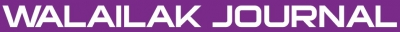### New Exact Solutions for Isothermal Magnetostatic Atmosphere Equations

Mohamed Tawfik ATTIA, Attala ELHANBALY, Mohamed Aly ABDOU

#### Abstract

Here, an extended, (G'/G)-expansion method with a computerized symbolic computation is used for constructing the exact travelling wave solutions for isothermal magnetostatic atmospheres equations. These equations depend on arbitrary functions that must be specified with choices of the different choice of the different arbitrary functions. The proposed method has been successfully used to obtain some exact travelling wave solutions for the Liouville and sinh-Poisson equations. The obtained travelling wave solutions are expressed by hyperbolic, triangular and exponential function. The solutions obtained via the propose method have many potential applications in physics.

doi:10.14456/WJST.2015.42

#### Keywords

Isothermal magnetostatic atmospheres equations, extended (G'/G)-expansion method, Liouville and sinh-Poisson equations, Liouville equation, travelling wave solutions

PDF

#### References

MJ Ablowitz and PA Clarkson. Soliton, Nonlinear Evolution Equations and Inverse Scattering. Cambridge University Press, New York, 1991, p. 1-516.

SA El-Wakil, SK El-Labany, MA Zahran and R Sabry. Modified extended tanh-function method and its applications to nonlinear equations. Appl. Math. Comput. 2005; 161, 403-12.

SA El-Wakil and MA Abdou. Modified extended tanh function method for solving nonlinear partial differential equations. Chaos Soliton. Fract. 2007; 31; 1256-64.

SA El-Wakil and MA Abdou. New exact travelling wave solutions of two nonlinear physical models. Nonlinear Anal. 2008; 68, 235-45.

LC Ping. General: (G'/G)-expansion method equivalent to extended tanh function method. Comm. Theor. Phys. 2009; 51, 985-8.

Y Peng, EV Krishnan and H Feng. Traveling wave-like solutions of the Zakharov-Kuznetsov equation with variable coefficients. Pramana J. Phys. 2008; 71, 49-55.

AM Wazwaz. New solitons and kinks solutions to the Sharma-Tasso-Olver equation. Appl. Math. Comput. 2007; 188, 1205-13.

A Malik, F Chand and SC Mishra. Exact travelling wave solutions of some nonlinear equations by (G'/G)-expansion method. Appl. Math. Comput. 2010; 216, 2596-612.

EME Zayed and KA Gepreel. Some applications of the (G'/G)-expansion method to non-linear partial differential equations. Appl. Math. Comput. 2009; 212, 1-13.

H Zhang. New application of the (G'/G)-expansion method. Comm. Nonlinear Sci. Numer. Simulat. 2009; 14, 3220-5.

HL Lu, XQ Liu and L Niu. A generalized (G'/G)-expansion method and its applications to nonlinear evolution equations. Appl. Math. Comput. 2010; 215, 3811-6.

LX Li and ML Wang. The (G'/G)-expansion method and travelling wave solutions for a higher-order nonlinear schrödinger equation. Appl. Math. Comput. 2009; 208, 440-5.

Y Uğurlu and D Kaya. Analytic method for solitary solutions of some partial differential equations. Phys. Lett. A 2007; 370, 251-9.

Z Li and X Zhang. New exact kink solutions and periodic form solutions for a generalized Zakharov-Kuznetsov equation with variable coefficients. Comm. Nonlinear Sci. Numer. Simulat. 2010; 15, 3418-22.

EM Abulwafa, MA Abdou and AA Mahmoud. The solution of nonlinear coagulation problem with mass loss. Chaos Soliton. Fract. 2006; 29, 313-30.

MA Abdou. Exact periodic wave solutions for some nonlinear evolution equations. Int. J. Nonlinear Sci. 2008; 5, 1-9.

MA Abdou and S Zhang. New periodic wave solutions via extended mapping method. Comm. Nonlinear Sci. Numer. Simulat. 2009; 14, 2-11.

SA El-Wakil, MA Madkour and MA Abdou. Application of Exp-function method for nonlinear evolution equations with variable coefficients. Phys. Lett. A 2007; 369, 62-9.

Y Zhou, M Wang and Y Wang. Periodic wave solutions to a coupled KdV equations with variable coefficients. Phys. Lett. A 2003; 308, 31-6.

MA Abdou. On the variational iteration method. Phys. Lett. A 2007; 366, 61-8.

SA El-Wakil, MA Abdou and A Hendi. New periodic wave solutions via Exp-function method. Phys. Lett. A 2008; 372, 830-40.

MA Abdou. Generalized solitary and periodic solutions for nonlinear partial differential equations by the Exp-function method. J. Nonlinear Dynam. 2008; 52, 1-9.

SA El-Wakil and MA Abdou. The extended mapping method and its applications for nonlinear evolutions equations. Phys. Lett. A 2006; 358, 275-82.

AH Khater, DK Callebaut and OH El-Kalaawy. Buacklund transformations and exact solutions for a nonlinear elliptic equation modelling isothermal magnetostatic atmosphere. IMA J. Appl. Math. 2000; 65, 97-108.

AH Khater, MA El-Attary, MF El-Sabbagh and DK Callebaut. Two-dimensional magnetohydrodynamic equilibria. Astrophys. Space Sci. 1988; 149, 217-23.

H Jafari, M Ghorbani and C Masood. Exact travelling wave solutions for isothermal magnetostatic atmospheres by fan subequation method. Abstr. Appl. Anal. 2012; 2012, Article ID 962789.

I Lerche and BC Low. Some nonlinear problems in astrophysics. Phys. D: Nonlinear Phenom. 1982; 4, 293-318.

H Jafari, N Kadkhoda and A Biswas. The (G'/G)-expansion method for solving of evolution equations from isothermal magnetostatic atmospheres. J. King Saud Univ. Sci. 2013; 25, 57-62

XH Wu and JH He. Solitary solutions, periodic solutions and compacton-like solutions using the Exp-function method. Comput. Math. Appl. 2007; 54, 966-86.

W Zwingmann. Theoretical study of onset conditions for solar eruptive processes. Solar Phys. 1987; 111, 309-31.

I Lerche and BC Low. On the equilibrium of a cylindrical plasma supported horizontally by magneticelds in uniform gravity. Solar Phys. 1980; 67, 229-43.

GM Webb. Isothermal magnetostatic atmospheres. II - Similarity solutions with current proportional to the magnetic potential cubed. Astrophys. J. 1988; 327, 933-49.

GM Webb and GP Zank. Application of the sine-Poisson equation in solar magnetostatics. Solar Phys. 1990; 127, 229-52.

### Refbacks

• There are currently no refbacks.Online ISSN: 2228-835X

http://wjst.wu.ac.th

Last updated: 20 June 2019